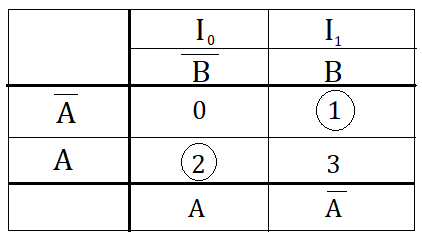## Posts

Showing posts with the label Design of Basic Gates using Multiplexer

### 2-Input XNOR Gate using 2:1 Multiplexer - Basic Gates design using MUXUsing Multiplexer any combinational logic circuit can be designed. Here you would see how to implement a 2-input  XNOR gate using a 2:1 multiplexer - Basic Gates design using MUX

### 2-Input XOR Gate using 2:1 Multiplexer - Basic Gates design using MUXUsing Multiplexer any combinational logic circuit can be designed. Here you would see how to implement a 2-input  XOR gate using a 2:1 multiplexer - Basic Gates design using MUX

### 2-Input NOR Gate using 2:1 Multiplexer - Basic Gates design using MUXUsing Multiplexer any combinational logic circuit can be designed. Here you would see how to implement a 2-input  NOR gate using a 2:1 multiplexer - Basic Gates design using MUX

### 2-Input NAND Gate using 2:1 Multiplexer - Basic Gates design using MUXMultiplexer can be used to design any combinational logic circuit. Here you would see how to implement a 2-input  NAND gate using a 2:1 multiplexer - Basic Gates design using MUX

### 2-Input OR Gate using 2:1 Multiplexer - Basic Gates design using MUXMultiplexer can be used to design any combinational logic circuit. Here you would see how to implement a 2-input  OR gate using a 2:1 multiplexer - Basic Gates design using MUX

### 2-Input AND Gate using 2:1 Multiplexer - Basic Gates design using MUXHere you would see how to implement a 2-input  AND gates using 2:1 multiplexer - Basic Gates design using MUX